# 3  Spatial data operations

## 3.1 Prerequisites

Let’s import the required packages:

import shapely
import geopandas as gpd
import numpy as np
import os
import rasterio
import rasterio.plot
import rasterio.merge
import rasterio.features
import scipy.ndimage
import scipy.stats
import richdem as rd

and load the sample data for this chapter:

nz = gpd.read_file('data/nz.gpkg')
src_elev = rasterio.open('data/elev.tif')
src_landsat = rasterio.open('data/landsat.tif')
src_grain = rasterio.open('data/grain.tif')

## 3.3 Spatial operations on vector data

### 3.3.1 Spatial subsetting

Spatial subsetting is the process of taking a spatial object and returning a new object containing only features that relate in space to another object. Analogous to attribute subsetting (covered in Section 2.3.1), subsets of GeoDataFrames can be created with square bracket ([) operator using the syntax x[y], where x is an GeoDataFrame from which a subset of rows/features will be returned, and y is a boolean Series. The difference is, that, in spatial subsetting y is created based on another geometry and using one of the binary geometry relation methods, such as .intersects (see Section 3.3.2), rather based on comparison based on ordinary columns.

To demonstrate spatial subsetting, we will use the nz and nz_height layers, which contain geographic data on the 16 main regions and 101 highest points in New Zealand, respectively (Figure 3.1 (a)), in a projected coordinate system. The following expression creates an object representing Canterbury (canterbury):

canterbury = nz[nz['Name'] == 'Canterbury']
canterbury
Name Island Land_area ... Median_income Sex_ratio geometry
10 Canterbury South 44504.499091 ... 30100 0.975327 MULTIPOLYGON (((1686901.914 535...

1 rows × 7 columns

Then, we use the .intersects method evaluate, for each of the nz_height points, whether they intersect with Canterbury. The result canterbury_height is a boolean Series with the “answers”:

sel = nz_height.intersects(canterbury.geometry.iloc)
sel
0      False
1      False
...
99     False
100    False
Length: 101, dtype: bool

Finally, we can subset nz_height using the latter series, resulting in the subset canterbury_height with only those points that intersect with Canterbury:

canterbury_height = nz_height[sel]
canterbury_height
t50_fid elevation geometry
4 2362630 2749 POINT (1378169.600 5158491.453)
5 2362814 2822 POINT (1389460.041 5168749.086)
... ... ... ...
93 2380300 2711 POINT (1654213.379 5349962.973)
94 2380308 2885 POINT (1654898.622 5350462.779)

70 rows × 3 columns

The result is shown in Figure 3.1 (b):

# Original
base = nz.plot(color='white', edgecolor='lightgrey')
nz_height.plot(ax=base, color='None', edgecolor='red');

# Subset (intersects)
base = nz.plot(color='white', edgecolor='lightgrey')
canterbury.plot(ax=base, color='lightgrey', edgecolor='darkgrey')
canterbury_height.plot(ax=base, color='None', edgecolor='red');

Like in attribute subsetting (Section 2.3.1), we are using a boolean series (sel), of the same length as the number of rows in the filtered table (nz_height), created based on a condition applied on itself. The difference is that the condition is not a comparison of attribute values, but an evaluation of a spatial relation. Namely, we evaluate whether each geometry of nz_height intersects with canterbury geometry, using the .intersects method.

Various topological relations can be used for spatial subsetting which determine the type of spatial relationship that features in the target object must have with the subsetting object to be selected. These include touches, crosses, or within, as we will see shortly in Section 3.3.2. The most commonly used method is .intersects, which we used in the last example. This is a ‘catch all’ topological relation, that will return features in the target that touch, cross or are within the source ‘subsetting’ object. As an example of another method, we can use .disjoint to obtain all points that do not intersect with Canterbury:

sel = nz_height.disjoint(canterbury.geometry.iloc)
canterbury_height2 = nz_height[sel]

The result is shown in Figure 3.2:

# Original
base = nz.plot(color='white', edgecolor='lightgrey')
nz_height.plot(ax=base, color='None', edgecolor='red');

# Subset (disjoint)
base = nz.plot(color='white', edgecolor='lightgrey')
canterbury.plot(ax=base, color='lightgrey', edgecolor='darkgrey')
canterbury_height2.plot(ax=base, color='None', edgecolor='red');

In case we need to subset according to several geometries at once, e.g., find out which points intersect with both Canterbury and Southland, we can dissolve the filtering subset, using .unary_union, before applying the .intersects (or any other) operator. For example, here is how we can subset the nz_height points which intersect with Canterbury or Southland:

canterbury_southland = nz[nz['Name'].isin(['Canterbury', 'Southland'])]
sel = nz_height.intersects(canterbury_southland.unary_union)
canterbury_southland_height = nz_height[sel]
canterbury_southland_height
t50_fid elevation geometry
0 2353944 2723 POINT (1204142.603 5049971.287)
4 2362630 2749 POINT (1378169.600 5158491.453)
... ... ... ...
93 2380300 2711 POINT (1654213.379 5349962.973)
94 2380308 2885 POINT (1654898.622 5350462.779)

71 rows × 3 columns

Alternatively, we can use .overlay to calculate the pairwise intersection between the canterbury_southland subset and nz_height. This approach is applicable for points (such as in the present example), where the intersection is the point itself, or when we are interested in line or polygon geometries (i.e., just the parts that intersect with the subsetting object) rather than complete geometries (such as in the example in Section 3.3.6)

nz_height.overlay(canterbury_southland)
t50_fid elevation Name ... Median_income Sex_ratio geometry
0 2353944 2723 Southland ... 29500 0.978507 POINT (1204142.603 5049971.287)
1 2362630 2749 Canterbury ... 30100 0.975327 POINT (1378169.600 5158491.453)
... ... ... ... ... ... ... ...
69 2380300 2711 Canterbury ... 30100 0.975327 POINT (1654213.379 5349962.973)
70 2380308 2885 Canterbury ... 30100 0.975327 POINT (1654898.622 5350462.779)

71 rows × 9 columns

The resulting subset is shown in Figure 3.3:

# Original
base = nz.plot(color='white', edgecolor='lightgrey')
nz_height.plot(ax=base, color='None', edgecolor='red');

# Subset by intersection with two polygons
base = nz.plot(color='white', edgecolor='lightgrey')
canterbury_southland.plot(ax=base, color='lightgrey', edgecolor='darkgrey')
canterbury_southland_height.plot(ax=base, color='None', edgecolor='red');

The next section further explores different types of spatial relation, also known as binary predicates (of which .intersects and .disjoint are two examples), that can be used to identify whether or not two features are spatially related or not.

### 3.3.2 Topological relations

Topological relations describe the spatial relationships between objects. “Binary topological relationships”, to give them their full name, are logical statements (in that the answer can only be True or False) about the spatial relationships between two objects defined by ordered sets of points (typically forming points, lines and polygons) in two or more dimensions (Egenhofer and Herring 1990, to add citation…). That may sound rather abstract and, indeed, the definition and classification of topological relations is based on mathematical foundations first published in book form in 1966 (Spanier 1995, to add citation…), with the field of algebraic topology continuing into the 21st century (Dieck 2008, to add citation…).

Despite their mathematical origins, topological relations can be understood intuitively with reference to visualizations of commonly used functions that test for common types of spatial relationships. Figure 3.4 shows a variety of geometry pairs and their associated relations. The third and fourth pairs in Figure 3.4 (from left to right and then down) demonstrate that, for some relations, order is important: while the relations equals, intersects, crosses, touches and overlaps are symmetrical, meaning that if x.relation(y) is true, y.relation(x) will also be true, relations in which the order of the geometries are important such as contains and within are not. Notice that each geometry pair has a “DE-9IM” string such as FF2F11212. DE-9IM strings describe the dimensionality (0=points, 1=lines, 2=polygons) of the pairwise intersections of the interior, boundary, and exterior, of two geometries (i.e., nine values of 0/1/2 encoded into a string). This is an advanced topic beyond the scope of this book, which can be useful to understand the difference between relation types, or define custom types of relations. See the DE-9IM strings section in Geocomputation with R (add citation…).Figure 3.4: Topological relations between vector geometries, inspired by Figures 1 and 2 in Egenhofer and Herring (1990). The relations for which the x.relation(y) is true are printed for each geometry pair, with x represented in pink and y represented in blue. The nature of the spatial relationship for each pair is described by the Dimensionally Extended 9-Intersection Model string.

In shapely, methods testing for different types of topological relations are known as “relationships”. geopandas provides warppers (with the same method name) which can be applied on multimple geometries at once (such as .intersects and .disjoint applied on all points in nz_height, see Section 3.3.1). To see how topological relations work in practice, let’s create a simple reproducible example, building on the relations illustrated in Figure 3.4 and consolidating knowledge of how vector geometries are represented from a previous chapter (Section 1.2.3 and Section 1.2.5):

points = gpd.GeoSeries([
shapely.Point(0.2,0.1),
shapely.Point(0.7,0.2),
shapely.Point(0.4,0.8)
])
line = gpd.GeoSeries([
shapely.LineString([(0.4,0.2), (1,0.5)])
])
poly = gpd.GeoSeries([
shapely.Polygon([(0,0), (0,1), (1,1), (1,0.5), (0,0)])
])

The sample dataset which we created is composed of three is GeoSeries: named points, line, and poly, which are visualized in Figure 3.5:

base = poly.plot(color='grey', edgecolor='red')
line.plot(ax=base, color='black', linewidth=7)
points.plot(ax=base, color='none', edgecolor='black')
for x, y, label in zip(points.x, points.y, [1,2,3]):
base.annotate(label, xy=(x, y), xytext=(3, 3), weight='bold', textcoords='offset points')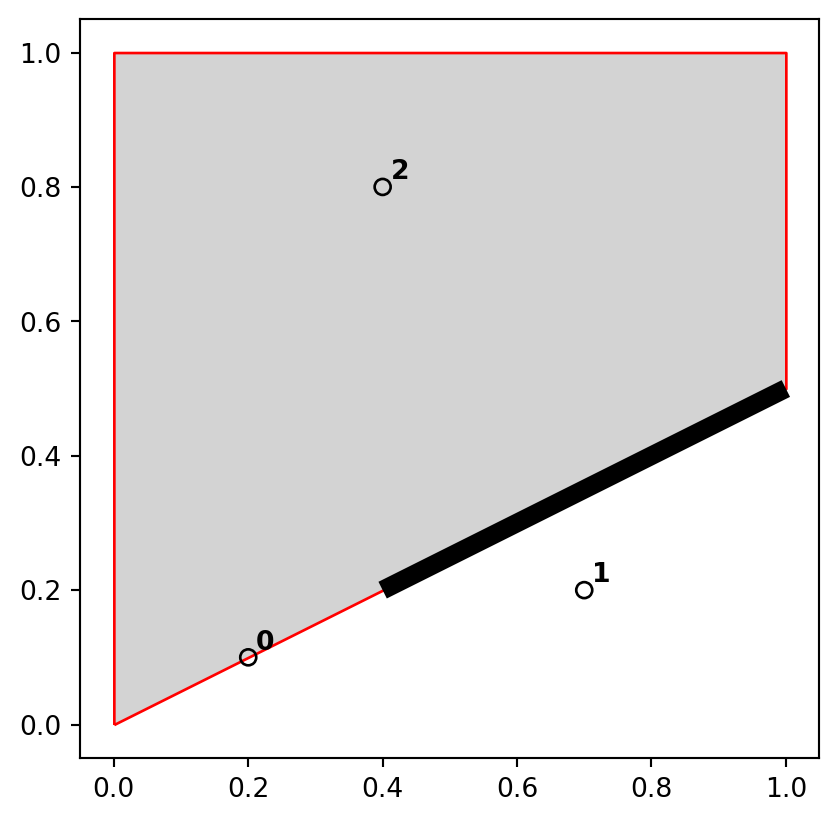Figure 3.5: Points, line and polygon objects arranged to illustrate topological relations

A simple query is: which of the points in points intersect in some way with polygon poly? The question can be answered by inspection (points 1 and 3 are touching and within the polygon, respectively). This question can be answered with the .intersects method, which reports whether or not each geometry in a GeoSeries (points) intersects with a single shapely geometry (poly.iloc).

points.intersects(poly.iloc)
0     True
1    False
2     True
dtype: bool

The result shown above is a boolean Series. Its contents should match your intuition: positive (True) results are returned for the first and third point, and a negative result (False) for the second are outside the polygon’s border. Each value in this Series represents a feature in the first input (points).

The last example, and all other earlier examples in this chapter, demonstrate the “many-to-one” mode of .intersects and analogous methods, where the relation is evaluated between each of several geometries in a GeoSeries/GeoDataFrame, and an individual shapely geometry. A second mode of those methods (not demonstrated here) is when both inputs are GeoSeries/GeoDataFrame objects. In such case, a “pairwise” evaluation takes place between geometries aligned by index (align=True, the default) or by position (align=False). For example, the expression nz.intersects(nz) returns a Series of 16 True values, indicating (unsurprisingly) that each geometry in nz intersects with itself.

A third mode is when we are interested in a “many-to-many” evaluation, i.e., obtaining a matrix of all pairwise combinations of geometries from two GeoSeries/GeoDataFrame objects. At the time of writing, there is no built-in method to do this in geopandas. However, the .apply method can be used to repeat a “many-to-one” evaluation over all geometries in the second layer,resulting in a matrix of pairwise results. We’ll create another GeoSeries with two polygons, named poly2, to demonstrate:

poly2 = gpd.GeoSeries([
shapely.Polygon([(0,0), (0,1), (1,1), (1,0.5), (0,0)]),
shapely.Polygon([(0,0), (1,0.5), (1,0), (0,0)])
])
points.apply(lambda x: poly2.intersects(x))
0 1
0 True True
1 False True
2 True False

The inputs for this example, points and poly2, are illustrated in Figure 3.6:

base = poly2.plot(color='none', edgecolor='red')
points.plot(ax=base, color='none', edgecolor='black')
for x, y, label in zip(points.x, points.y, [1,2,3]):
base.annotate(label, xy=(x, y), xytext=(3, 3), weight='bold', textcoords="offset points")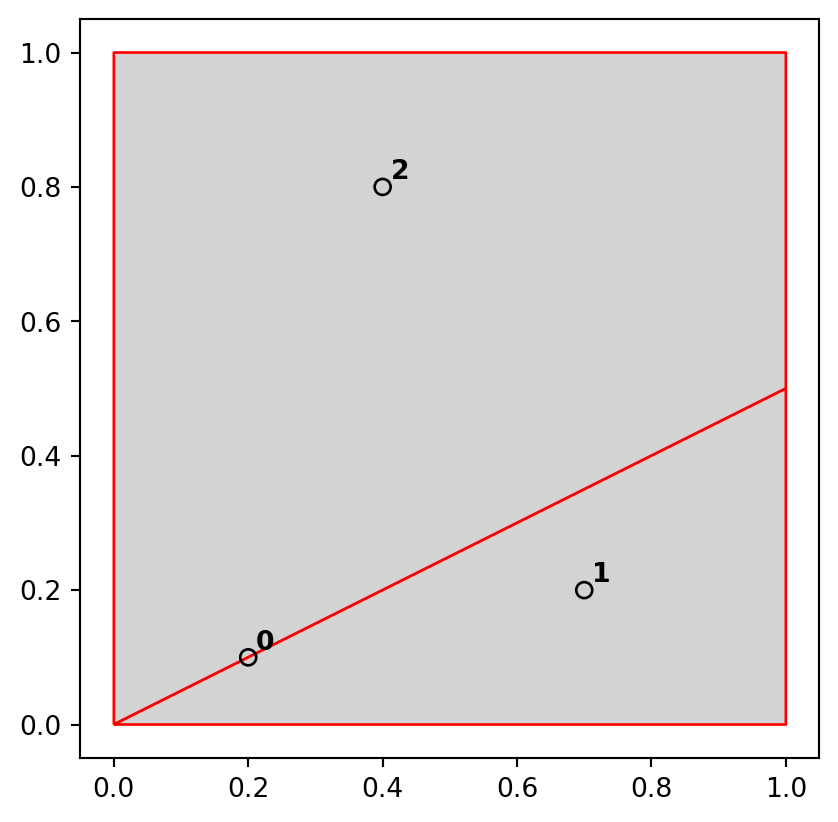Figure 3.6: Inputs for demonstrating the evaluation of all pairwise intersection relations between three points (points) and two polygons (poly2)

And here is how we can use .apply to get the intersection relations matrix. The result is a DataFrame, where each row represents a points geometry and each column represents a poly geometry. We can see that the first point intersects with both polygons, while the second and third points intersect with one of the polygons each:

points.apply(lambda x: poly2.intersects(x))
0 1
0 True True
1 False True
2 True False

A more natural way to work with this result is perhaps as an array:

points.apply(lambda x: poly2.intersects(x)).to_numpy()
array([[ True,  True],
[False,  True],
[ True, False]])

The .intersects method returns True even in cases where the features just touch: intersects is a ‘catch-all’ topological operation which identifies many types of spatial relation, as illustrated in Figure 3.4. More restrictive questions include which points lie within the polygon, and which features are on or contain a shared boundary with it? These can be answered as follows:

points.within(poly.iloc)
0    False
1    False
2     True
dtype: bool
points.touches(poly.iloc)
0     True
1    False
2    False
dtype: bool

Note that although the first point touches the boundary polygon, it is not within it; the third point is within the polygon but does not touch any part of its border. The opposite of .intersects is .disjoint, which returns only objects that do not spatially relate in any way to the selecting object:

points.disjoint(poly.iloc)
0    False
1     True
2    False
dtype: bool

Another useful type of relation is “within distance”, where we detect features that intersect with the target buffered by particular distance. Buffer distance determines how close target objects need to be before they are selected. This can be done by literally buffering (Section 1.2.5) the target geometry, and evaluating intersection (.intersects). Another way is to calculate the distances and compare them to the distance threshold:

points.distance(poly.iloc) < 0.2
0    True
1    True
2    True
dtype: bool

Note that although the second point is more than 0.2 units of distance from the nearest vertex of poly, it is still selected when the distance is set to 0.2. This is because distance is measured to the nearest edge, in this case the part of the the polygon that lies directly above point 2 in Figure Figure 3.4. We can verify the actual distance between the second point and the polygon is 0.13, as follows:

points.iloc.distance(poly.iloc)
0.13416407864998736

### 3.3.3 Spatial joining

Joining two non-spatial datasets relies on a shared ‘key’ variable, as described in Section 2.3.3. Spatial data joining applies the same concept, but instead relies on spatial relations, described in the previous section. As with attribute data, joining adds new columns to the target object (the argument x in joining functions), from a source object (y).

The process is illustrated by the following example: imagine you have ten points randomly distributed across the Earth’s surface and you ask, for the points that are on land, which countries are they in? Implementing this idea in a reproducible example will build your geographic data handling skills and show how spatial joins work. The starting point is to create points that are randomly scattered over the Earth’s surface (Figure 3.7 (a)):

np.random.seed(11)       ## set seed for reproducibility
bb = world.total_bounds  ## the world's bounds
x = np.random.uniform(low=bb, high=bb, size=10)
y = np.random.uniform(low=bb, high=bb, size=10)
random_points = gpd.points_from_xy(x, y, crs=4326)
random_points = gpd.GeoDataFrame({'geometry': random_points})
random_points
geometry
0 POINT (-115.10291 36.78178)
1 POINT (-172.98891 -71.02938)
... ...
8 POINT (159.05039 -34.99599)
9 POINT (126.28622 -62.49509)

10 rows × 1 columns

The scenario illustrated in Figure 3.7 shows that the random_points object (top left) lacks attribute data, while the world (top right) has attributes, including country names shown for a sample of countries in the legend. Spatial joins are implemented with x.sjoin(y), as illustrated in the code chunk below. The output is the random_joined object which is illustrated in Figure 3.7 (c). Before creating the joined dataset, we use spatial subsetting to create world_random, which contains only countries that contain random points, to verify the number of country names returned in the joined dataset should be four (see the top right panel of Figure 3.7 (b)):

world_random = world[world.intersects(random_points.unary_union)]
world_random
iso_a2 name_long continent ... lifeExp gdpPercap geometry
4 US United States North America ... 78.841463 51921.984639 MULTIPOLYGON (((-171.73166 63.7...
18 RU Russian Federation Europe ... 70.743659 25284.586202 MULTIPOLYGON (((-180.00000 64.9...
52 ML Mali Africa ... 57.007000 1865.160622 MULTIPOLYGON (((-11.51394 12.44...
159 AQ Antarctica Antarctica ... NaN NaN MULTIPOLYGON (((-180.00000 -89....

4 rows × 11 columns

Now we can do the spatial join:

random_joined = random_points.sjoin(world, how='left')
random_joined
geometry index_right iso_a2 ... pop lifeExp gdpPercap
0 POINT (-115.10291 36.78178) 4.0 US ... 318622525.0 78.841463 51921.984639
1 POINT (-172.98891 -71.02938) NaN NaN ... NaN NaN NaN
... ... ... ... ... ... ... ...
8 POINT (159.05039 -34.99599) NaN NaN ... NaN NaN NaN
9 POINT (126.28622 -62.49509) NaN NaN ... NaN NaN NaN

10 rows × 12 columns

The input points and countries, the illustration of intersecting countries, and the join result, are shown in Figure 3.7:

# Random points
base = world.plot(color='white', edgecolor='lightgrey')
random_points.plot(ax=base, color='None', edgecolor='red');

# World countries intersecting with the points
base = world.plot(color='white', edgecolor='lightgrey')
world_random.plot(ax=base, column='name_long');

# Points with joined country names
base = world.plot(color='white', edgecolor='lightgrey')
random_joined.geometry.plot(ax=base, color='grey')
random_joined.plot(ax=base, column='name_long', legend=True);

### 3.3.4 Non-overlapping joins

Sometimes two geographic datasets do not touch but still have a strong geographic relationship. The datasets cycle_hire and cycle_hire_osm, provide a good example. Plotting them shows that they are often closely related but they do not touch, as shown in Figure 3.8:

base = cycle_hire.plot(edgecolor='blue', color='none')
cycle_hire_osm.plot(ax=base, edgecolor='red', color='none');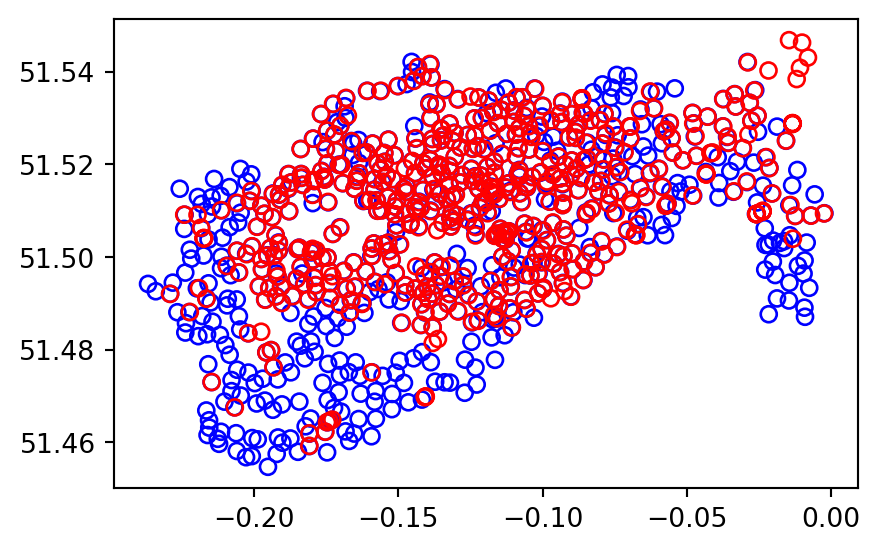Figure 3.8: The spatial distribution of cycle hire points in London based on official data (blue) and OpenStreetMap data (red).

We can check if any of the points are the same by creating a pairwise boolean matrix of .intersects relations, then evaluating whether any of the values in it is True. Note that the .to_numpy method is applied to go from a DataFrame to a numpy array, for which .any gives a global rather than a row-wise summary, which is what we want in this case:

m = cycle_hire.geometry.apply(
lambda x: cycle_hire_osm.geometry.intersects(x)
)
m.to_numpy().any()
False

Imagine that we need to join the capacity variable in cycle_hire_osm ('capacity') onto the official ‘target’ data contained in cycle_hire, which looks as follows:

cycle_hire
id name area nbikes nempty geometry
0 1 River Street Clerkenwell 4 14 POINT (-0.10997 51.52916)
1 2 Phillimore Gardens Kensington 2 34 POINT (-0.19757 51.49961)
... ... ... ... ... ... ...
740 776 Abyssinia Close Clapham Junction 10 10 POINT (-0.16703 51.46033)
741 777 Limburg Road Clapham Common 7 11 POINT (-0.16530 51.46192)

742 rows × 6 columns

This is when a non-overlapping join is needed. Spatial join (gpd.sjoin) along with buffered geometries can be used to do that. This is demonstrated below, using a threshold distance of 20 $$m$$. Note that we transform the data to a projected CRS (27700) to use real buffer distances, in meters.

crs = 27700
cycle_hire_buffers = cycle_hire.copy().to_crs(crs)
cycle_hire_buffers.geometry = cycle_hire_buffers.buffer(20)
z = gpd.sjoin(
cycle_hire_buffers,
cycle_hire_osm.to_crs(crs)
)
z
id name_left area ... capacity cyclestreets_id description
0 1 River Street Clerkenwell ... 9.0 NaN NaN
1 2 Phillimore Gardens Kensington ... 27.0 NaN NaN
... ... ... ... ... ... ... ...
729 765 Ranelagh Gardens Fulham ... 29.0 NaN NaN
737 773 Tallis Street Temple ... 14.0 NaN NaN

458 rows × 12 columns

Note that the number of rows in the joined result is greater than the target. This is because some cycle hire stations in cycle_hire_buffers have multiple matches in cycle_hire_osm. To aggregate the values for the overlapping points and return the mean, we can use the aggregation methods learned in Section 2.3.2, resulting in an object with the same number of rows as the target. We also go back from buffers to points using .centroid:

z = z[['id', 'capacity', 'geometry']] \
.dissolve(by='id', aggfunc='mean') \
.reset_index()
z.geometry = z.centroid
z
id geometry capacity
0 1 POINT (531203.517 182832.066) 9.0
1 2 POINT (525208.067 179391.922) 27.0
... ... ... ...
436 765 POINT (524652.998 175817.001) 29.0
437 773 POINT (531435.032 180916.010) 14.0

438 rows × 3 columns

The capacity of nearby stations can be verified by comparing a plot of the capacity of the source cycle_hire_osm data, with the join results in the new object z (Figure 3.9):

# Input
fig, ax = plt.subplots(1, 1, figsize=(6, 3))
cycle_hire_osm.plot(column='capacity', legend=True, ax=ax);
# Join result
fig, ax = plt.subplots(1, 1, figsize=(6, 3))
z.plot(column='capacity', legend=True, ax=ax);

### 3.3.5 Spatial aggregation

As with attribute data aggregation, spatial data aggregation condenses data: aggregated outputs have fewer rows than non-aggregated inputs. Statistical aggregating functions, such as mean, average, or sum, summarise multiple values of a variable, and return a single value per grouping variable. Section 2.3.2 demonstrated how the .groupby method, combined with summary functions such as .sum, condense data based on attribute variables. This section shows how grouping by spatial objects can be acheived using spatial joins combined with non-spatial aggregation.

Returning to the example of New Zealand, imagine you want to find out the average height of high points in each region. It is the geometry of the source (nz) that defines how values in the target object (nz_height) are grouped. This can be done in three steps:

1. Figuring out which nz region each nz_height point falls in—using gpd.sjoin
2. Summarizing the average elevation per region—using .groupby and .mean
3. Joining the result back to nz—using pd.merge

First, we ‘attach’ the region classification of each point, using spatial join (Section 3.3.3). Note that we are using the minimal set of columns required: the geometries (for the spatial join to work), the point elevation (to later calculate an average), and the region name (to use as key when joining the results back to nz). The result tells us which nz region each elevation point falls in:

nz_height2 = gpd.sjoin(
nz_height[['elevation', 'geometry']],
nz[['Name', 'geometry']],
how='left'
)
nz_height2
elevation geometry index_right Name
0 2723 POINT (1204142.603 5049971.287) 12 Southland
1 2820 POINT (1234725.325 5048309.302) 11 Otago
... ... ... ... ...
99 2720 POINT (1822262.592 5650428.656) 2 Waikato
100 2732 POINT (1822492.184 5650492.304) 2 Waikato

101 rows × 4 columns

Second, we calculate the average elevation, using ordinary (non-spatial) aggregation (Section 2.3.2). This result tells us the average elevation of all nz_height points located within each nz region:

nz_height2 = nz_height2.groupby('Name')[['elevation']].mean()
nz_height2
elevation
Name
Canterbury 2994.600000
Manawatu-Wanganui 2777.000000
... ...
Waikato 2734.333333
West Coast 2889.454545

7 rows × 1 columns

The third and final step is joining the averages back to the nz layer:

nz2 = pd.merge(nz[['Name', 'geometry']], nz_height2, on='Name', how='left')
nz2
Name geometry elevation
0 Northland MULTIPOLYGON (((1745493.196 600... NaN
1 Auckland MULTIPOLYGON (((1803822.103 590... NaN
... ... ... ...
14 Nelson MULTIPOLYGON (((1624866.279 541... NaN
15 Marlborough MULTIPOLYGON (((1686901.914 535... 2720.0

16 rows × 3 columns

We now have create the nz_height4 layer, which gives the average nz_height elevation value per polygon. The result is shown in Figure 3.10. Note that the missing_kwds part determines the style of geometries where the symbology attribute (elevation) is missing, because there were no nz_height points overlapping with them. The default is to omit them, which is usually not what we want. With {'color':'none','edgecolor':'black'}, those polygons are shown with black outline and no fill.

nz2.plot(
column='elevation',
legend=True,
cmap='Blues', edgecolor='black',
missing_kwds={'color': 'none', 'edgecolor': 'black'}
);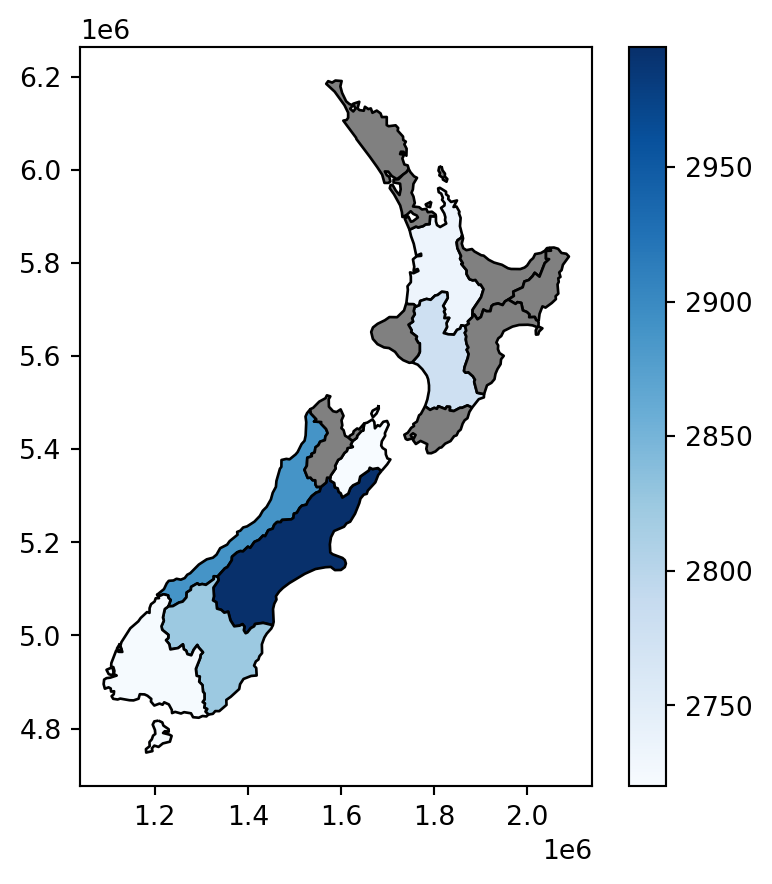Figure 3.10: Average height of the top 101 high points across the regions of New Zealand

### 3.3.6 Joining incongruent layers

Spatial congruence is an important concept related to spatial aggregation. An aggregating object (which we will refer to as y) is congruent with the target object (x) if the two objects have shared borders. Often this is the case for administrative boundary data, whereby larger units—such as Middle Layer Super Output Areas (MSOAs) in the UK, or districts in many other European countries—are composed of many smaller units.

Incongruent aggregating objects, by contrast, do not share common borders with the target (Qiu, Zhang, and Zhou 2012, to add citation…). This is problematic for spatial aggregation (and other spatial operations) illustrated in Figure 3.11: aggregating the centroid of each sub-zone will not return accurate results. Areal interpolation overcomes this issue by transferring values from one set of areal units to another, using a range of algorithms including simple area weighted approaches and more sophisticated approaches such as ‘pycnophylactic’ methods (Tobler 1979, to add citation…).

To demonstrate, we will create a “synthetic” layer comprising a regular grid of rectangles of size $$100\times100$$ $$km$$, covering the extent of the nz layer:

xmin, ymin, xmax, ymax = nz.total_bounds
res = 100000
cols = list(range(int(np.floor(xmin)), int(np.ceil(xmax+res)), res))
rows = list(range(int(np.floor(ymin)), int(np.ceil(ymax+res)), res))
rows.reverse()
polygons = []
for x in cols:
for y in rows:
polygons.append(
shapely.Polygon([(x,y), (x+res, y), (x+res, y-res), (x, y-res)])
)
grid = gpd.GeoDataFrame({'geometry': polygons}, crs=nz.crs)
sel = grid.intersects(shapely.box(*nz.total_bounds))
grid = grid[sel]
grid['id'] = grid.index
grid
geometry id
0 POLYGON ((1090143.000 6248536.0... 0
1 POLYGON ((1090143.000 6148536.0... 1
... ... ...
157 POLYGON ((1990143.000 4948536.0... 157
158 POLYGON ((1990143.000 4848536.0... 158

150 rows × 2 columns

The grid layer which we have just created is shown in Figure 3.11:

base = grid.plot(color='none', edgecolor='grey')
nz.plot(ax=base, column='Population', edgecolor='black', legend=True, cmap='viridis_r');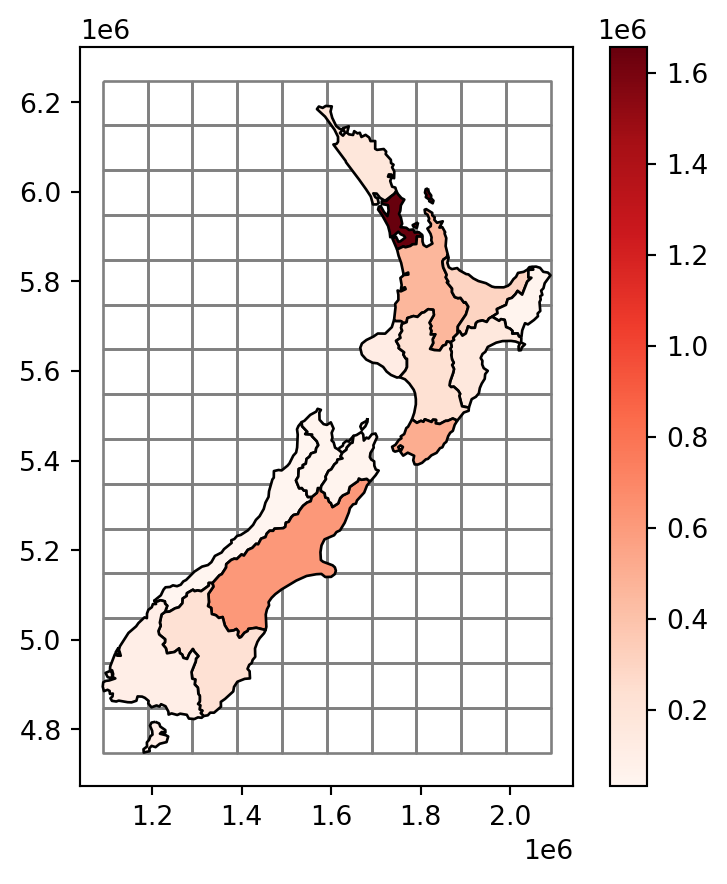Figure 3.11: The nz layer and a regular grid of rectangles

Our goal, now, is to “transfer” the Population attribute (Figure 3.11) to the rectangular grid polygons, which is an example of a join between incongruent layers. To do that, we basically need to calculate–for each grid cell—the weighted sum of the population in nz polygons coinciding with that cell. The weights in the weighted sum calculation are the ratios between the area of the coinciding “part” out of the entire nz polygon. That is, we (inevitably) assume that the population in each nz polygon is equally distributed across space, therefore a partial nz polygon contains the respective partial population size.

We start with calculating the entire area of each nz polygon, as follows, using the .area method (Section 1.2.7):

nz['area'] = nz.area
nz
Name Island Land_area ... Sex_ratio geometry area
0 Northland North 12500.561149 ... 0.942453 MULTIPOLYGON (((1745493.196 600... 1.289058e+10
1 Auckland North 4941.572557 ... 0.944286 MULTIPOLYGON (((1803822.103 590... 4.911565e+09
... ... ... ... ... ... ... ...
14 Nelson South 422.195242 ... 0.925967 MULTIPOLYGON (((1624866.279 541... 4.080754e+08
15 Marlborough South 10457.745485 ... 0.957792 MULTIPOLYGON (((1686901.914 535... 1.046485e+10

16 rows × 8 columns

Next, we use the .overlay method to calculate the pairwise intersections between nz and grid. As a result, we now have a layer where each nz polygon was “split” according to the grid polygons, hereby named nz_grid:

nz_grid = nz.overlay(grid)
nz_grid = nz_grid[['id', 'area', 'Population', 'geometry']]
nz_grid
id area Population geometry
0 64 1.289058e+10 175500.0 POLYGON ((1586362.965 6168009.0...
1 80 1.289058e+10 175500.0 POLYGON ((1590143.000 6162776.6...
... ... ... ... ...
108 87 4.080754e+08 51400.0 POLYGON ((1649908.695 5455398.2...
109 87 1.046485e+10 46200.0 MULTIPOLYGON (((1678688.086 545...

110 rows × 4 columns

Figure 3.12 illustrates the effect of .overlay:

nz_grid.plot(color='none', edgecolor='black');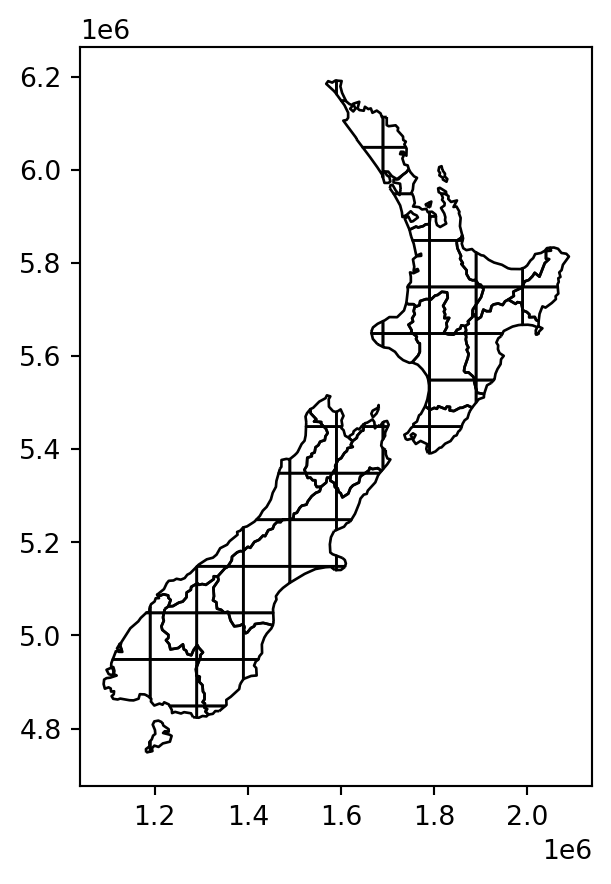Figure 3.12: The pairwise intersections of nz and grid, calculated with .overlay

Next, we calculate the areas of the intersections, into a new attribute we name 'area_sub'. If an nz polygon was completely within a single grid polygon, then area_sub is going to be equal to area; otherwise, it is going to be smaller:

nz_grid['area_sub'] = nz_grid.area
nz_grid
id area Population geometry area_sub
0 64 1.289058e+10 175500.0 POLYGON ((1586362.965 6168009.0... 3.231015e+08
1 80 1.289058e+10 175500.0 POLYGON ((1590143.000 6162776.6... 4.612641e+08
... ... ... ... ... ...
108 87 4.080754e+08 51400.0 POLYGON ((1649908.695 5455398.2... 1.716260e+07
109 87 1.046485e+10 46200.0 MULTIPOLYGON (((1678688.086 545... 4.526248e+08

110 rows × 5 columns

The resulting layer nz_grid, which the area_sub attribute, is shown in Figure 3.13:

base = grid.plot(color='none', edgecolor='grey')
nz_grid.plot(ax=base, column='area_sub', edgecolor='black', legend=True, cmap='viridis_r');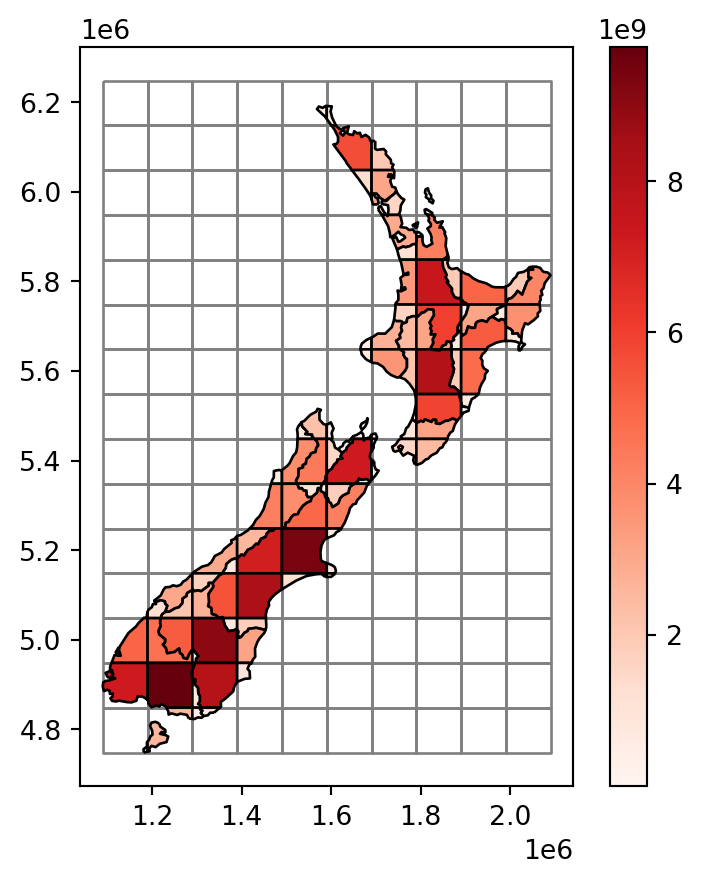Figure 3.13: The areas of pairwise intersections in the nz_grid layer

Note that each of the “intersections” still holds the Population attribute of its “origin” feature of nz, i.e., each portion of the nz area is associated with the original complete population count for that area. The real population size of each nz_grid feature, however, is smaller, or equal, depending on the geographic area proportion that it occupies out of the original nz feature. To make the “correction”, we first calculate the ratio (area_prop) and then multiply it by the population. The new (lowercase) attribute population now has the correct estimate of population sizes in nz_grid:

nz_grid['area_prop'] = nz_grid['area_sub'] / nz_grid['area']
nz_grid['population'] = nz_grid['Population'] * nz_grid['area_prop']
nz_grid
id area Population ... area_sub area_prop population
0 64 1.289058e+10 175500.0 ... 3.231015e+08 0.025065 4398.897141
1 80 1.289058e+10 175500.0 ... 4.612641e+08 0.035783 6279.925114
... ... ... ... ... ... ... ...
108 87 4.080754e+08 51400.0 ... 1.716260e+07 0.042057 2161.752203
109 87 1.046485e+10 46200.0 ... 4.526248e+08 0.043252 1998.239223

110 rows × 7 columns

What is left to be done is to sum (see Section 2.3.2) the population in all parts forming the same grid cell:

nz_grid = nz_grid.groupby('id')['population'].sum().reset_index()
nz_grid
id population
0 11 67.533590
1 12 15339.996965
... ... ...
55 149 31284.910446
56 150 129.326331

57 rows × 2 columns

and join (see Section 2.3.3) them back to the grid layer. Note that many of the grid cells have “No Data” for population, because they have no intersection with nz at all (Figure 3.11):

grid = pd.merge(grid, nz_grid[['id', 'population']], on='id', how='left')
grid
geometry id population
0 POLYGON ((1090143.000 6248536.0... 0 NaN
1 POLYGON ((1090143.000 6148536.0... 1 NaN
... ... ... ...
148 POLYGON ((1990143.000 4948536.0... 157 NaN
149 POLYGON ((1990143.000 4848536.0... 158 NaN

150 rows × 3 columns

The final result grid, with the incongruently-joined population attribute from nz, is shown in Figure 3.14:

base = grid.plot(column='population', edgecolor='black', legend=True, cmap='viridis_r');
nz.plot(ax=base, color='none', edgecolor='grey', legend=True);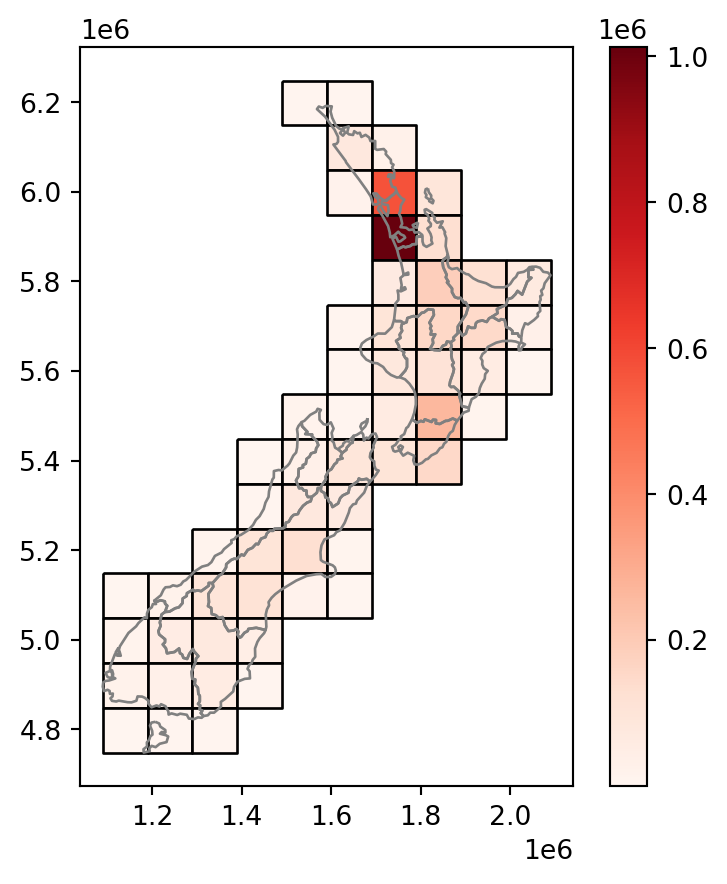Figure 3.14: The nz layer and a regular grid of rectangles: final result

We can demonstrate that, expectedly, the summed population in nz and grid is identical, even though the geometry is different (since we created grid to completely cover nz):

nz['Population'].sum()
4787200.0
grid['population'].sum()
4787199.999999998

The procedure in this section is known as an area-weighted interpolation of a spatially extensive (e.g., population) variable. An area-weighted interpolation of a spatially intensive variable (e.g., population density) is almost identical, except that we would have to calculate the weighted .mean rather than .sum, to preserve the average rather than the sum.

### 3.3.7 Distance relations

While topological relations are binary—a feature either intersects with another or does not—distance relations are continuous. The distance between two objects is calculated with the .distance method. The method is applied on a GeoSeries (or a GeoDataFrame), with the argument being an individual shapely geometry. The result is a Series of pairwise distances. (The .distance method syntax is thus analogous to the topological evaluations methods, such as .intersects (Section 3.3.2), only that the results are numeric rather than boolean Series.

To illustrate the .distance method, let’s take the three highest point in New Zealand:

nz_highest = nz_height \
.sort_values(by='elevation', ascending=False) \
.iloc[:3, :]
nz_highest
t50_fid elevation geometry
64 2372236 3724 POINT (1369317.630 5169132.284)
63 2372235 3717 POINT (1369512.866 5168235.616)
67 2372252 3688 POINT (1369381.942 5168761.875)

and the geographic centroid of the Canterbury region (canterbury, created in Section 3.3.1):

canterbury_centroid = canterbury.centroid.iloc

Now we apply .distance to calculate the distances from each of the three elevation points to Canterbury:

nz_highest.distance(canterbury_centroid)
64    115539.995747
63    115390.248038
67    115493.594066
dtype: float64

To obtain a distance matrix, i.e., a pairwise set of distances between all combinations of features in objects x and y, we need to use the .apply method (analogous to the way we created the .intersects boolean matrix in Section 3.3.2). To illustrate, let’s now take two regions in nz, Otago and Canterbury, represented by the object co:

sel = nz['Name'].str.contains('Canter|Otag')
co = nz[sel]
co
Name Island Land_area ... Sex_ratio geometry area
10 Canterbury South 44504.499091 ... 0.975327 MULTIPOLYGON (((1686901.914 535... 4.532656e+10
11 Otago South 31186.309188 ... 0.951169 MULTIPOLYGON (((1335204.789 512... 3.190356e+10

2 rows × 8 columns

The distance matrix (technically speaking, a DataFrame) d between each of the first three elevation points, and the two regions, is then obtained as follows. In plain language, we take the geometry from each each row in nz_height.iloc[:3,:], and apply the .distance method on co with that row as the argument:

d = nz_height.iloc[:3, :].apply(lambda x: co.distance(x.geometry), axis=1)
d
10 11
0 123537.158269 15497.717252
1 94282.773074 0.000000
2 93018.560814 0.000000

Note that the distance between the second and third features in nz_height and the second feature in co is zero. This demonstrates the fact that distances between points and polygons refer to the distance to any part of the polygon: the second and third points in nz_height are in Otago, which can be verified by plotting them (Figure 3.15):

base = co.iloc[].plot(color='none')
nz_height.iloc[1:3, :].plot(ax=base, color='none', edgecolor='black');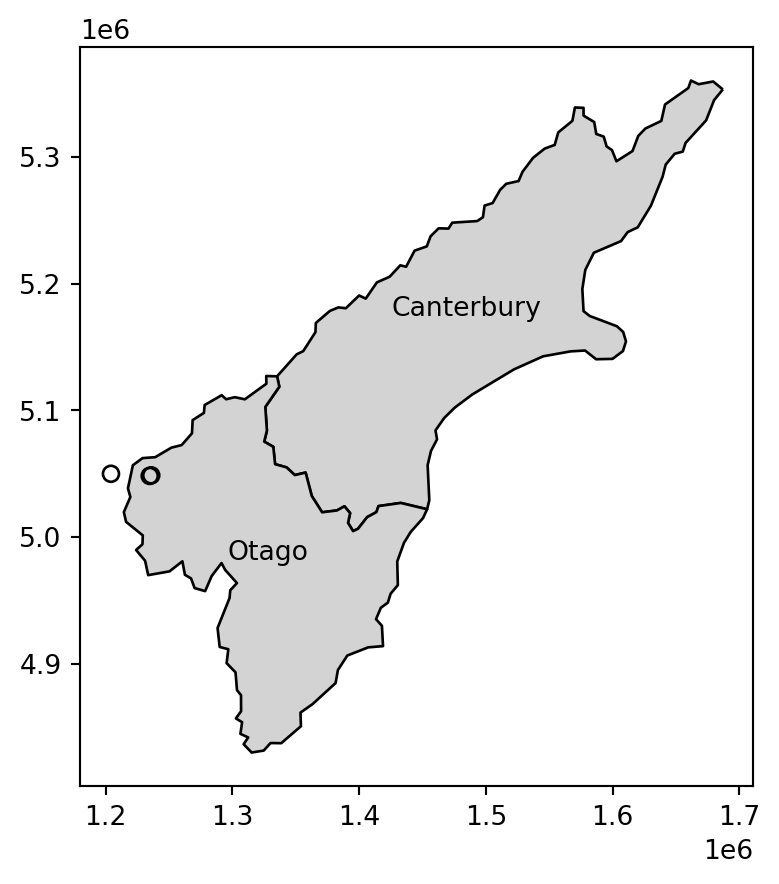Figure 3.15: The second and third nz_height points, and the Otago ploygon from nz

## 3.4 Spatial operations on raster data

### 3.4.1 Spatial subsetting

The previous chapter (Section Section 2.4) demonstrated how to retrieve values associated with specific cell IDs, or row and column combinations, from a raster. Raster values can also be extracted by location (coordinates) and other spatial objects. To use coordinates for subsetting, we can use the .sample method of a rasterio file connection object, combined with a list of coordinate tuples. The methods is demonstrated below to find the value of the cell that covers a point located at coordinates of (0.1,0.1) in elev. The returned object is a generator:

src_elev.sample([(0.1, 0.1)])
<generator object sample_gen at 0x7fb32a8b9640>

In case we want all values at once, we can apply list. Since there was just one point, the result is just one extracted value, in this case 16:

list(src_elev.sample([(0.1, 0.1)]))
[array(, dtype=uint8)]

We can use the same technique to extract the values of multiple points at once. For example, here we extract the raster values at two points, (0.1,0.1) and (1.1,1.1). The resulting values are 16 and 6:

list(src_elev.sample([(0.1, 0.1), (1.1, 1.1)]))
[array(, dtype=uint8), array(, dtype=uint8)]

The location of the two sample points on top of the elev.tif raster is illustrated in Figure 3.16:

fig, ax = plt.subplots()
rasterio.plot.show(src_elev, ax=ax)
gpd.GeoSeries([shapely.Point(0.1, 0.1)]).plot(color='black', ax=ax)
gpd.GeoSeries([shapely.Point(1.1, 1.1)]).plot(color='black', ax=ax);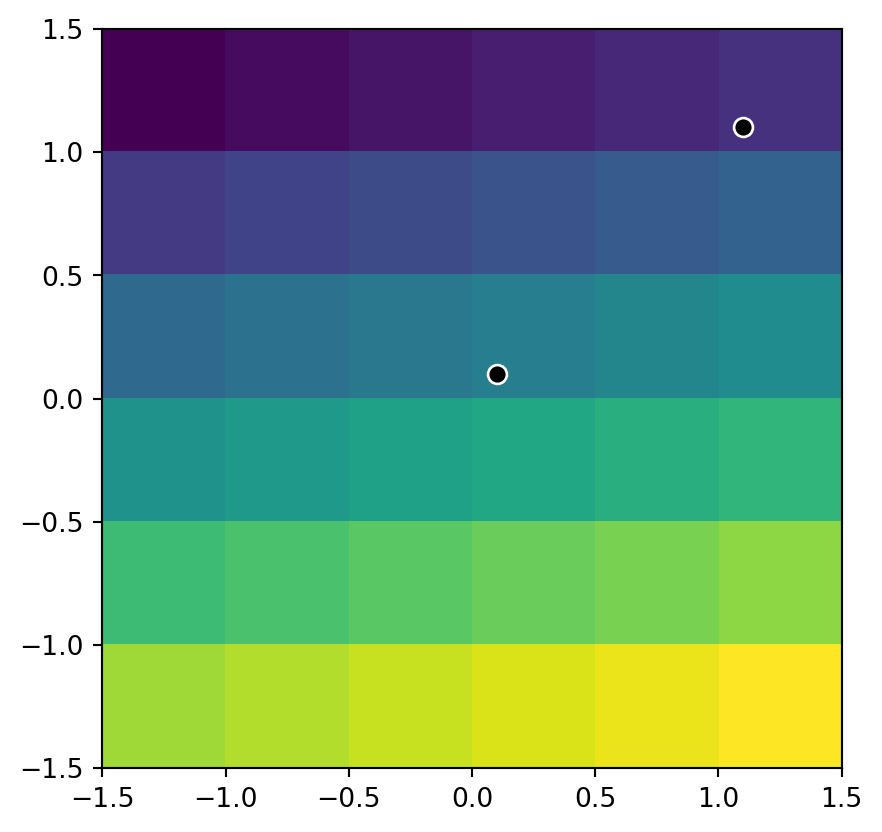Figure 3.16: The elev.tif raster, and two points where we extract its values

We elaborate on the plotting technique used to display the points and the raster in Section 8.3.4. We will also introduce a more user-friendly and general method to extract raster values to points, using the rasterstats package, in Section 5.4.1.

Another common use case of spatial subsetting is using a boolean mask, based on another raster with the same extent and resolution, or the original one, as illustrated in Figure 3.17. To do that, we “erase” the values in the array of one raster, according to another corresponding “mask” raster. For example, let us read (Section 1.3.2) the elev.tif raster values into an array named elev (we will also close the connection, to avoid too many open connections in this chapter which may lead to an error):

elev = src_elev.read(1)
src_elev.close()
elev
array([[ 1,  2,  3,  4,  5,  6],
[ 7,  8,  9, 10, 11, 12],
[13, 14, 15, 16, 17, 18],
[19, 20, 21, 22, 23, 24],
[25, 26, 27, 28, 29, 30],
[31, 32, 33, 34, 35, 36]], dtype=uint8)

and create a correspinding random boolean mask named mask:

np.random.seed(1)
mask
array([[False, False,  True,  True, False, False],
[False, False, False,  True,  True, False],
[ True, False, False,  True,  True, False],
[ True,  True,  True, False,  True,  True],
[False,  True,  True,  True, False,  True],
[ True,  True, False, False, False, False]])

In the code chunk above, we have created a mask object called mask with values randomly assigned to True and False. Next, suppose that we want to keep those values of elev which are False in mask (i.e., they are not masked). In other words, we want to mask elev with mask. The result is stored in a copy named elev1. To be able to store np.nan in the raster, we also need to convert it to float (see Section 2.4.2):

elev1 = elev.copy()
elev1 = elev1.astype('float64')
elev1
array([[ 1.,  2., nan, nan,  5.,  6.],
[ 7.,  8.,  9., nan, nan, 12.],
[nan, 14., 15., nan, nan, 18.],
[nan, nan, nan, 22., nan, nan],
[25., nan, nan, nan, 29., nan],
[nan, nan, 33., 34., 35., 36.]])

The result is shown in Figure 3.17:

rasterio.plot.show(elev);
rasterio.plot.show(elev1);

The above approach can be also used to replace some values (e.g., expected to be wrong) with np.nan:

elev1 = elev.copy()
elev1 = elev1.astype('float64')
elev1[elev1 < 20] = np.nan
elev1
array([[nan, nan, nan, nan, nan, nan],
[nan, nan, nan, nan, nan, nan],
[nan, nan, nan, nan, nan, nan],
[nan, 20., 21., 22., 23., 24.],
[25., 26., 27., 28., 29., 30.],
[31., 32., 33., 34., 35., 36.]])

These operations are in fact Boolean local operations, since we compare, cell-wise, two rasters. The next subsection explores these and related operations in more detail.

### 3.4.2 Map algebra

The term ‘map algebra’ was coined in the late 1970s to describe a “set of conventions, capabilities, and techniques” for the analysis of geographic raster and (although less prominently) vector data (Tomlin 1994, to add citation…). In this context, we define map algebra more narrowly, as operations that modify or summarise raster cell values, with reference to surrounding cells, zones, or statistical functions that apply to every cell.

Map algebra operations tend to be fast, because raster datasets only implicitly store coordinates, hence the old adage “raster is faster but vector is corrector”. The location of cells in raster datasets can be calculated by using its matrix position and the resolution and origin of the dataset (stored in the raster metadata, Section 1.3.2). For the processing, however, the geographic position of a cell is barely relevant as long as we make sure that the cell position is still the same after the processing. Additionally, if two or more raster datasets share the same extent, projection and resolution, one could treat them as matrices for the processing.

Map algebra (or cartographic modeling with raster data) divides raster operations into four subclasses (Tomlin 1990, to add citation…), with each working on one or several grids simultaneously:

• Local or per-cell operations (Section 3.4.3)
• Focal or neighborhood operations. Most often the output cell value is the result of a $$3 \times 3$$ input cell block (Section 3.4.4)
• Zonal operations are similar to focal operations, but the surrounding pixel grid on which new values are computed can have irregular sizes and shapes (Section 3.4.5)
• Global or per-raster operations; that means the output cell derives its value potentially from one or several entire rasters (Section 3.4.6)

This typology classifies map algebra operations by the number of cells used for each pixel processing step and the type of the output. For the sake of completeness, we should mention that raster operations can also be classified by discipline such as terrain, hydrological analysis, or image classification. The following sections explain how each type of map algebra operations can be used, with reference to worked examples.

### 3.4.3 Local operations

Local operations comprise all cell-by-cell operations in one or several layers. Raster algebra is a classical use case of local operations—this includes adding or subtracting values from a raster, squaring and multipling rasters. Raster algebra also allows logical operations such as finding all raster cells that are greater than a specific value (e.g., 5 in our example below). Local operations are applied using the numpy array operations syntax, as demonstrated below.

First, let’s take the array of elev.tif raster values, which we already read earlier (Section 3.4.1):

elev
array([[ 1,  2,  3,  4,  5,  6],
[ 7,  8,  9, 10, 11, 12],
[13, 14, 15, 16, 17, 18],
[19, 20, 21, 22, 23, 24],
[25, 26, 27, 28, 29, 30],
[31, 32, 33, 34, 35, 36]], dtype=uint8)

Now, any element-wise array operation can be applied using numpy arithmetic or conditional operators and functions, comprising local raster operations in spatial analysis terminology. For example:

elev + elev
array([[ 2,  4,  6,  8, 10, 12],
[14, 16, 18, 20, 22, 24],
[26, 28, 30, 32, 34, 36],
[38, 40, 42, 44, 46, 48],
[50, 52, 54, 56, 58, 60],
[62, 64, 66, 68, 70, 72]], dtype=uint8)

Note that some functions and operators automatically change the data type to accomodate the resulting values, while other operators do not, potentially resulting in overflow (i.e., incorrect values for results beyond the data type range, such as 255 for int8). For example, elev**2 (elev squared) results in overflow:

elev**2
array([[  1,   4,   9,  16,  25,  36],
[ 49,  64,  81, 100, 121, 144],
[169, 196, 225,   0,  33,  68],
[105, 144, 185, 228,  17,  64],
[113, 164, 217,  16,  73, 132],
[193,   0,  65, 132, 201,  16]], dtype=uint8)

To accomodate the results, we can, for instance, transform elev to the standard int64 data type before applying the operator. Now we get correct results:

elev.astype(int)**2
array([[   1,    4,    9,   16,   25,   36],
[  49,   64,   81,  100,  121,  144],
[ 169,  196,  225,  256,  289,  324],
[ 361,  400,  441,  484,  529,  576],
[ 625,  676,  729,  784,  841,  900],
[ 961, 1024, 1089, 1156, 1225, 1296]])

Figure 3.18 demonstrates the result of the last two examples (elev+elev and elev.astype(int)**2), and two other ones (np.log(elev) and elev>5):

rasterio.plot.show(elev + elev, cmap='Oranges');
rasterio.plot.show(elev.astype(int)**2, cmap='Oranges');
rasterio.plot.show(np.log(elev), cmap='Oranges');
rasterio.plot.show(elev > 5, cmap='Oranges');

Another good example of local operations is the classification of intervals of numeric values into groups such as grouping a digital elevation model into low (class 1), middle (class 2) and high elevations (class 3). Here, we assign the raster values in the ranges 012, 1224 and 2436 are reclassified to take values 1, 2 and 3, respectively.

recl = elev.copy()
recl[(elev > 0)  & (elev <= 12)] = 1
recl[(elev > 12) & (elev <= 24)] = 2
recl[(elev > 24) & (elev <= 36)] = 3

The reclassified result is shown in Figure 3.19:

rasterio.plot.show(elev, cmap='Oranges');
rasterio.plot.show(recl, cmap='Oranges');

The calculation of the Normalized Difference Vegetation Index (NDVI) is a well-known local (pixel-by-pixel) raster operation. It returns a raster with values between -1 and 1; positive values indicate the presence of living plants (mostly > 0.2). NDVI is calculated from red and near-infrared (NIR) bands of remotely sensed imagery, typically from satellite systems such as Landsat or Sentinel. Vegetation absorbs light heavily in the visible light spectrum, and especially in the red channel, while reflecting NIR light, explaining the NVDI formula (?eq-ndvi):

$NDVI=\frac{NIR-Red} {NIR+Red}$

where $$NIR$$ is the near-infrared band and $$Red$$ is the red band.

Let’s calculate NDVI for the multispectral Landsat satellite file (landsat.tif) of the Zion National Park:

landsat = src_landsat.read()
src_landsat.close()
nir = landsat
red = landsat
ndvi = (nir-red)/(nir+red)

Convert values >1 to “No Data”:

ndvi[ndvi>1] = np.nan

When plotting an RGB image using the rasterio.plot.show function, the function assumes that:

• Values are in the range [0,1] for floats, or [0,255] for integers (otherwise clipped)
• The order of bands is RGB

To “prepare” the multi-band raster for rasterio.plot.show, we therefore reverse the order of the first three bands (to go from B-G-R-NIR to R-G-B), using the [:3] slice to select the first three bands and then the [::-1] slice to reverse the bands order, and divide by the maximum to set the maximum value at 1:

landsat_rgb = landsat[:3][::-1] / landsat.max()

The result is shown in the right panel of Figure 3.20:

rasterio.plot.show(landsat_rgb, cmap='RdYlGn');
rasterio.plot.show(ndvi, cmap='Greens');

### 3.4.4 Focal operations

While local functions operate on one cell, though possibly from multiple layers, focal operations take into account a central (focal) cell and its neighbors. The neighborhood (also named kernel, filter or moving window) under consideration is typically of $$3 \times 3$$ cells (that is, the central cell and its eight surrounding neighbors), but can take on any other (not necessarily rectangular) shape as defined by the user. A focal operation applies an aggregation function to all cells within the specified neighborhood, uses the corresponding output as the new value for the the central cell, and moves on to the next central cell (Figure 3.21). Other names for this operation are spatial filtering and convolution .Figure 3.21: Input raster (left) and resulting output raster (right) due to a focal operation—finding the minimum value in $$3 \times 3$$ moving windows.

In Python, the scipy.ndimage package has a comprehensive collection of functions to perform filtering of numpy arrays, such as:

In this group of functions, we define the shape of the moving window with either one of:

• size—a single number (e.g., 3), or tuple (e.g., (3,3)), implying a filter of those dimensions
• footprint—a boolean array, representing both the window shape and the identity of elements being included

In addition to specific built-in filters,

• convolve applies the sum function after multiplying by a custom weights array
• generic_filter makes it possible to pass any custom function, where the user can specify any type of custom window-based calculation.

For example, here we apply the minimum filter with window size of 3 on elev. As a result, we now have a new array elev_min, where each value is the minimum in the corresponding $$3 \times 3$$ neighborhood in elev:

elev_min = scipy.ndimage.minimum_filter(elev, size=3)
elev_min
array([[ 1,  1,  2,  3,  4,  5],
[ 1,  1,  2,  3,  4,  5],
[ 7,  7,  8,  9, 10, 11],
[13, 13, 14, 15, 16, 17],
[19, 19, 20, 21, 22, 23],
[25, 25, 26, 27, 28, 29]], dtype=uint8)

Special care should be given to the edge pixels. How should they be calculated? The scipy.ndimage filtering functions give several options through the mode parameter (see the documentation of any filtering function, such as scipy.ndimage.median_filter, for the definition of each mode):

• reflect (the default)
• constant
• nearest
• mirror
• wrap

Sometimes artificially extending raster edges is considered unsuitable. In other words, we may wish the resulting raster to contain pixel values with “complete” windows only, for example to have a uniform sample size or because values in all directions matter (such as in topographic calculations). There is no specific option not to extend edges in scipy.ndimage. However, to get the same effect, the edges of the filtered array can be assigned with np.nan, in a number of rows and columns according to filter size. For example, when using a filter of size=3, the outermost “layer” of pixels may be assigned with np.nan, reflecting the fact that these pixels have incomplete $$3 \times 3$$ neighborhoods:

elev_min = elev_min.astype(float)
elev_min[:, [0, -1]] = np.nan
elev_min[[0, -1], :] = np.nan
elev_min
array([[nan, nan, nan, nan, nan, nan],
[nan,  1.,  2.,  3.,  4., nan],
[nan,  7.,  8.,  9., 10., nan],
[nan, 13., 14., 15., 16., nan],
[nan, 19., 20., 21., 22., nan],
[nan, nan, nan, nan, nan, nan]])

We can quickly check if the output meets our expectations. In our example, the minimum value has to be always the upper left corner of the moving window (remember we have created the input raster by row-wise incrementing the cell values by one starting at the upper left corner).

Focal functions or filters play a dominant role in image processing. Low-pass or smoothing filters use the mean function to remove extremes. In the case of categorical data, we can replace the mean with the mode, which is the most common value.

To demonstrate applying a mode filter, let’s read the small sample categorical raster grain.tif:

grain = src_grain.read(1)
grain
array([[1, 0, 1, 2, 2, 2],
[0, 2, 0, 0, 2, 1],
[0, 2, 2, 0, 0, 2],
[0, 0, 1, 1, 1, 1],
[1, 1, 1, 2, 1, 1],
[2, 1, 2, 2, 0, 2]], dtype=uint8)

There is no built-in filter function for a mode filter in scipy.ndimage, but we can use the scipy.ndimage.generic_filter function along with a custom filtering function, internally utilizing scipy.stats.mode:

grain_mode = scipy.ndimage.generic_filter(
grain,
lambda x: scipy.stats.mode(x.flatten()),
size=3
)
grain_mode = grain_mode.astype(float)
grain_mode[:, [0, -1]] = np.nan
grain_mode[[0, -1], :] = np.nan
grain_mode
/tmp/ipykernel_253/45878578.py:3: FutureWarning: Unlike other reduction functions (e.g. skew, kurtosis), the default behavior of mode typically preserves the axis it acts along. In SciPy 1.11.0, this behavior will change: the default value of keepdims will become False, the axis over which the statistic is taken will be eliminated, and the value None will no longer be accepted. Set keepdims to True or False to avoid this warning.
lambda x: scipy.stats.mode(x.flatten()),
array([[nan, nan, nan, nan, nan, nan],
[nan,  0.,  0.,  0.,  2., nan],
[nan,  0.,  0.,  0.,  1., nan],
[nan,  1.,  1.,  1.,  1., nan],
[nan,  1.,  1.,  1.,  1., nan],
[nan, nan, nan, nan, nan, nan]])

By contrast, high-pass filters accentuate features. The line detection Laplace and Sobel filters might serve as an example here.

Terrain processing, the calculation of topographic characteristics such as slope, aspect and flow directions, relies on focal functions. These are specialized functions that are beyond the scope of scipy.ndimage, found in more narrow-scope packages. For example, the TerrainAttribute function from package richdem can be used to calculate common terrain metrics, specified through the attrib argument, namely:

• slope_riserun
• slope_percentage
• slope_degrees
• slope_radians
• aspect
• curvature
• planform_curvature
• profile_curvature

Full description of the richdem package is beyond the scope of this book. However, you can get an idea of how it works in the following code section. You can see that richdem has its own data structure for rasters (also numpy-based, like rasterio), and consequently its own import and export functions. In the example, we import the srtm_32612.tif file (which we are going to create in Section 6.9), calculates slope (in decimal degrees), and exports the result to a new file srtm_32612_slope.tif:

srtm = rd.LoadGDAL('output/srtm_32612.tif')
srtm_slope = rd.TerrainAttribute(srtm, 'slope_degrees')
rd.SaveGDAL('output/srtm_32612_slope.tif', srtm_slope)

Figure 3.23 shows the result, using our more familiar plotting methods from rasterio. The code section is relatively long due to the workaround to create a color key (see Section 8.3.3) and removing “No Data” flag values from the arrays so that the color key does not include them:

# Input (DEM)
src_srtm = rasterio.open('output/srtm_32612.tif')
srtm[srtm == src_srtm.nodata] = np.nan
fig, ax = plt.subplots()
i = ax.imshow(srtm, cmap='Spectral')
rasterio.plot.show(src_srtm, cmap='Spectral', ax=ax)
fig.colorbar(i, ax=ax);

# Output (slope)
src_srtm_slope = rasterio.open('output/srtm_32612_slope.tif')
srtm_slope[srtm_slope == src_srtm_slope.nodata] = np.nan
fig, ax = plt.subplots()
i = ax.imshow(srtm_slope, cmap='Spectral')
rasterio.plot.show(src_srtm_slope, cmap='Spectral', ax=ax)
fig.colorbar(i, ax=ax);

### 3.4.5 Zonal operations

Just like focal operations, zonal operations apply an aggregation function to multiple raster cells. However, a second raster, usually with categorical values, defines the zonal filters (or ‘zones’) in the case of zonal operations, as opposed to a predefined neighborhood window in the case of focal operation presented in the previous section. Consequently, raster cells defining the zonal filter do not necessarily have to be neighbors. Our grain.tif raster is a good example, as illustrated in Figure 1.21: different grain sizes are spread irregularly throughout the raster. Finally, the result of a zonal operation is a summary table grouped by zone, which is why this operation is also known as zonal statistics in the GIS world. This is in contrast to focal operations (Section 3.4.4) which return a raster object.

To demonstrate, let’s get back to the grain.tif and elev.tif rasters. To calculate zonal statistics, we use the arrays with raster values, which we already imported earlier:

grain
array([[1, 0, 1, 2, 2, 2],
[0, 2, 0, 0, 2, 1],
[0, 2, 2, 0, 0, 2],
[0, 0, 1, 1, 1, 1],
[1, 1, 1, 2, 1, 1],
[2, 1, 2, 2, 0, 2]], dtype=uint8)
elev
array([[ 1,  2,  3,  4,  5,  6],
[ 7,  8,  9, 10, 11, 12],
[13, 14, 15, 16, 17, 18],
[19, 20, 21, 22, 23, 24],
[25, 26, 27, 28, 29, 30],
[31, 32, 33, 34, 35, 36]], dtype=uint8)

Our interntion is to calculate the average (or any other summary function, for that matter) of elevation in each zone defined by grain values. To do that, first we first obtain the unique values defining the zones, using np.unique:

np.unique(grain)
array([0, 1, 2], dtype=uint8)

Now, we can use dictionary conprehension to “split” the elev array into separate one-dimensional arrays with values per grain group, with keys being the unique grain values:

z = {i: elev[grain == i] for i in np.unique(grain)}
z
{0: array([ 2,  7,  9, 10, 13, 16, 17, 19, 20, 35], dtype=uint8),
1: array([ 1,  3, 12, 21, 22, 23, 24, 25, 26, 27, 29, 30, 32], dtype=uint8),
2: array([ 4,  5,  6,  8, 11, 14, 15, 18, 28, 31, 33, 34, 36], dtype=uint8)}

At this stage, we can expand the dictionary comprehension expression to calculate the mean elevation associated with each grain size class. Namely, instead of placing the elevation values (elev[grain==i]) into the dictionary values, we place their (rounded) mean (elev[grain==i].mean().round(1)):

z = {i: elev[grain == i].mean().round(1) for i in np.unique(grain)}
z
{0: 14.8, 1: 21.2, 2: 18.7}

This returns the statistics for each category, here the mean elevation for each grain size class. For example, the mean elevation in pixels characterized by grain size 0 is 14.8, and so on.

### 3.4.6 Global operations and distances

Global operations are a special case of zonal operations with the entire raster dataset representing a single zone. The most common global operations are descriptive statistics for the entire raster dataset such as the minimum or maximum—we already discussed those in Section 2.4.2.

Aside from that, global operations are also useful for the computation of distance and weight rasters. In the first case, one can calculate the distance from each cell to specific target cells or vector geometries. For example, one might want to compute the distance to the nearest coast (see Section 5.7). We might also want to consider topography, that means, we are not only interested in the pure distance but would like also to avoid the crossing of mountain ranges when going to the coast. To do so, we can weight the distance with elevation so that each additional altitudinal meter “prolongs” the Euclidean distance (this is beyond the scope of the book). Visibility and viewshed computations also belong to the family of global operations (also beyond the scope of the book).

### 3.4.7 Map algebra counterparts in vector processing

Many map algebra operations have a counterpart in vector processing . Computing a distance raster (global operation) while only considering a maximum distance (logical focal operation) is the equivalent to a vector buffer operation (Section 4.3.3). Reclassifying raster data (either local or zonal function depending on the input) is equivalent to dissolving vector data (Section 4.3.7). Overlaying two rasters (local operation), where one contains “No Data” values representing a mask, is similar to vector clipping (Section Section 4.3.5). Quite similar to spatial clipping is intersecting two layers (Section 3.3.1, Section 3.3.6). The difference is that these two layers (vector or raster) simply share an overlapping area. However, be careful with the wording. Sometimes the same words have slightly different meanings for raster and vector data models. While aggregating polygon geometries means dissolving boundaries, for raster data geometries it means increasing cell sizes and thereby reducing spatial resolution. Zonal operations dissolve the cells of one raster in accordance with the zones (categories) of another raster dataset using an aggregating function.

### 3.4.8 Merging rasters

Suppose we would like to compute the NDVI (see Section 3.4.3), and additionally want to compute terrain attributes from elevation data for observations within a study area. Such computations rely on remotely sensed information. The corresponding imagery is often divided into scenes covering a specific spatial extent (i.e., “tiles”), and frequently, a study area covers more than one scene. Then, we would need to merge (also known as “mosaic”) the scenes covered by our study area. In case when all scenes are “aligned” (i.e., share the same origin and resolution), this can be thought of as simply gluing them into one big raster; otherwise, all scenes are resampled (see Section 4.4.4) to the grid defined by the first scene.

For example, let us merge digital elevation data from two SRTM elevation tiles, for Austria ('aut.tif') and Switzerland ('ch.tif'). Merging can be done using function rasterio.merge.merge, which accepts a list of raster file connections, and returns the new ndarray and a “transform”, representing the resulting mosaic:

src_1 = rasterio.open('data/aut.tif')
src_2 = rasterio.open('data/ch.tif')
out_image, out_transform = rasterio.merge.merge([src_1, src_2])

Both inputs and the result are shown in Figure 3.24:

rasterio.plot.show(src_1);
rasterio.plot.show(src_2);
rasterio.plot.show(out_image, transform=out_transform);

By default in rasterio.merge.merge (method='first'), areas of overlap retain the value of the first raster. Other possible methods are:

• 'last'—Value of the last raster
• 'min'—Minimum value
• 'max'—Maximum value

When dealing with non-overlapping tiles, such as aut.tif and ch.tif (above), the method argument has no practical effect. However, it becomes relevant when we want to combine spectral imagery from scenes that were taken on different dates. The above four options for method do not cover the commonly required scenario when we would like to compute the mean value—for example to calculate a seasonal average NDVI image from a set of partially overlapping satellite images (such as Landsat). An alternative worflow to rasterio.merge.merge, for calculating a mosaic as well as “averaging” any overlaps, is to go through two steps:

• Resampling all scenes into a common “global” grid (Section 4.4.4), thereby producing a series of “matching” rasters (with the area surrounding each scene set as “No Data”)
• Averaging the rasters through raster algebra (Section 3.4.3), using np.mean(m,axis=0) or np.nanmean(m,axis=0) (depending whether we prefer to ignore “No Data” or not), where m is the multi-band array, which would return a single-band array of averages

## 3.5 Exercises

• Write a function which accepts and array and an int specifying the number of rows/columns to erase along an array edges. The function needs to return the modified array with np.nan values along its edges.